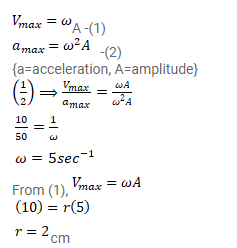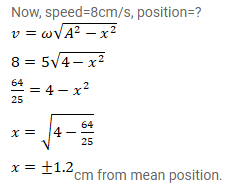# The maximum speed and acceleration of a particle executing simple harmonic motion

Question:

The maximum speed and acceleration of a particle executing simple harmonic motion are $10 \mathrm{~cm} / \mathrm{s}$ and $50^{\mathrm{cm}} / \mathrm{s}^{2}$. Find the position(s) of the particle when the speed is $8 \mathrm{~cm} / \mathrm{s}$.

Solution: